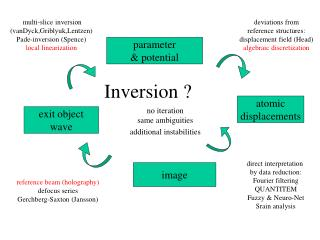DownloadDownload PresentationInversion ?

# Inversion ?

Télécharger la présentation## Inversion ?

- - - - - - - - - - - - - - - - - - - - - - - - - - - E N D - - - - - - - - - - - - - - - - - - - - - - - - - - -
##### Presentation Transcript

1. multi-slice inversion (vanDyck,Griblyuk,Lentzen) Pade-inversion (Spence) local linearization deviations from reference structures: displacement field (Head) algebraic discretization parameter & potential Inversion ? atomic displacements exit object wave no iteration same ambiguities additional instabilities direct interpretation by data reduction: Fourier filtering QUANTITEM Fuzzy & Neuro-Net Srain analysis image reference beam (holography) defocus series Gerchberg-Saxton (Jansson)

2. Data lost?Additional data? phases linearity reference beam defocus series Scattering process Imaging process lattices & bonds shape & orientation displacement field inelastic spectra 3d-2d projection atom positions

3. regularization physically motivated Assumption: complex amplitudes are regular Cauchy relations: Ja/Jx = a.Jf/Jy Ja/Jy = -a.Jf/Jx Linear inversion: t(x+1,y)-2t(x,y)+t(x-1,y)=0 t(x,y+1)-2t(x,y)+t(x,y-1)=0

4. Direct & Inverse: black box gedankenexperiment thickness local orientation structure & defects composition microscope g output f input operator A wave image theory, hypothesis, model of scattering and imaging if unique & stable inverse A-1 exists ill-posed & insufficient data => least square direct: g=A<f experiment, measurement invers 1.kind: f=A-1<g parameter determination invers 2.kind: A=g\$f -1 identification, interpretation a priori knowledge intuition & induction additional data

5. perfect crystal: f = e2piAt fo distorted object: Jf/Jz= pi(sA+Dxy+b) f f, Jf/Jz continuous at boundaries b = J(gu)/Jz displacement field J(ff*)/Jz = e-pmt energy conservation fo fo fg i,j-1 i,j i,j+1 i-1,j forward wave equation =>f(i+1,j) backward energy conservation =>b(i-1,j) i+1,j i,j-1 i,j i,j+1 solve equations of perfect crystal, discretize wave equations and boundary conditions =>algebraic equation system of f, b at all nodes (i,j,k) and SQge2pigu(i,j,k) = 0